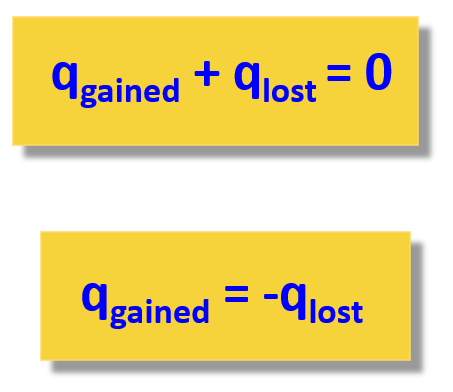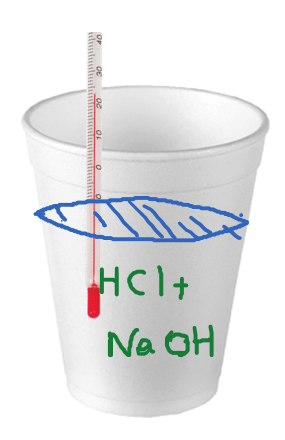## General Chemistry

A calorimeter is a device for measuring heat flow. One application of calorimetry is measuring the enthalpy of a reaction.

So, how does heat become enthalpy?

Remember, the formula for enthalpy change is:

ΔH = ΔE + ΔPV

Now, many reactions are carried out under constant pressure as most of the time, we set up the experiment without worrying about the pressure. Therefore, if we plug in the expression ΔE = q +w in the enthalpy change equation, we get that the enthalpy change (ΔH) is equal to the heat when the pressure is constant:Once we have this settled, the rest of measuring the enthalpy of a reaction is similar to what we discussed about heat capacity and heat flow from one object to another. Remember, the key to solving problems in thermochemistry is the assumption that the heat is not lost, and it only flows from one object to the other:So, for calorimetry, we treat the reaction as one object or a system and the calorimeter as another object. The calorimeter can also be viewed as the surroundings. This does not change the principle that the heat flows between the reaction and the calorimeter.

qrxn + qcal = 0

The heat transfer to/from the calorimeter is determined by its temperature change and the heat capacity:

qcal = Ccal x ΔT

The heat capacity of the calorimeter is determined experimentally and is already known when measuring the heat/enthalpy of a reaction.

# Coffee-cup Calorimeter

Although the calorimeters are designed for highly accurate measurements of heat transfers, a simple “coffee-cup” or “styrofoam-cup” calorimeter is often used in general chemistry laboratories. The styrofoam is good enough insulator of heat and it serves the purpose of creating a closed system. So, the  heat released or absorbed by the reaction will raise or lower the temperature of the water in the solution. This allows for calculating the heat of the solution, which is numerically equal to the heat of the reaction:

qsolution = – qrxn

qsolution is calculated as:

qsoln = Cs m ΔT

where Cs is the specific heat capacity of water (4.18 J/g oC), m is the mass of the solution, and ΔT is the temperature change of the solution.

For example,

When a 50.0 mL of 1.0 M HCl solution is mixed with 50.0 mL of 1.0 M NaOH in a coffee-cup calorimeter, the temperature of the resultant solution increases from 22.0 to 28.0 oC. Calculate the enthalpy change for the reaction in kJ/mol HCl assuming that the density of all solutions is 1 g/mL.We have an exothermic reaction because the temperature of the solution went up, and assuming the calorimeter does not lose any heat, we write that:

qrxn = – qsolution

Next, calculate the qsoln:

qsoln = Cs m ΔT

We have the Cs of water (4.18 J/g oC), the ΔT = 28.0 – 22.0 = 6.00 oC. The mass of the solution is the sum of the two solutions, and because each solution is 50.0 g, it is 100. g.

Therefore,

qsoln = 4.18 J/g oC m ΔT

${q_{{\rm{soln}}}}\; = \;mC\Delta T\; = \,{\rm{100}}{\rm{.}}\;\cancel{{\rm{g}}}{\rm{ \times 4}}{\rm{.18}}\;\frac{{\rm{J}}}{{\cancel{{\rm{g}}}\,\cancel{{^{\rm{o}}{\rm{C}}}}}}\, \times 6.00\,\cancel{{^{\rm{o}}{\rm{C}}}}\; = \;2.51\, \times \,{10^3}\,{\rm{J}}$

This corresponds to 2.51 kJ:

${\rm{2}}{\rm{.51}}\,{\rm{ \times }}\,{\rm{1}}{{\rm{0}}^{\rm{3}}}\,{\rm{J}}\,{\rm{ \times }}\;\frac{{{\rm{1}}\;{\rm{kJ}}}}{{{\rm{1000}}\,{\rm{J}}}}\;{\rm{ = }}\;{\rm{2}}{\rm{.51}}\;{\rm{kJ}}$

The enthalpy change of the reaction is equal to negative 2.51 kJ and corresponds to these specific amounts of reactants. However, the problem asks to determine the ΔH per one mol of HCl!

So, we need to first calculate how many moles of HCl were in the 50.0 mL of 1.0 M HCl solution. For this, we use the molarity formula:

${\rm{n}}\;{\rm{(HCl)}}\;{\rm{ = }}\,{\rm{1}}\;\frac{{{\rm{mol}}}}{{\cancel{{\rm{L}}}}}\,{\rm{ \times }}\,{\rm{50}}{\rm{.0}}\,\cancel{{{\rm{mL}}}}\;{\rm{ \times }}\,\frac{{{\rm{1}}\,\cancel{{\rm{L}}}}}{{{\rm{1000}}\;\cancel{{{\rm{mL}}}}}}\;{\rm{ = }}\,{\rm{0}}{\rm{.0500}}\;{\rm{mol}}$

So, 0.0500 mol of HCl releases 2.51 kJ heat, therefore, 1 mole of HCl would release:

2.51 kJ/0.0500 mol = 50.2 kJ/mol

And this is the enthalpy change of the reaction per one mol of HCl: ΔH = -50.2 kJ/mol

Check Also